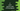# C programming example to print the source code of the current program### C programming example to print the source code of the current program :

This is a commonly asked interview question for C. In this tutorial, you will learn how to print the source code of the current program. The output of the program is the program itself :

### C program :

``````#include <stdio.h>

int main(){
FILE *fp;
char c;

fp = fopen(__FILE__,"r");

do{
c = getc(fp);
putchar(c);
}while(c != EOF);

fclose(fp);
return 0;
}
``````

It will print :

``````#include <stdio.h>

int main(){
FILE *fp;
char c;

fp = fopen(__FILE__,"r");

do{
c = getc(fp);
putchar(c);
}while(c != EOF);

fclose(fp);
return 0;
}
``````

### How it works :

The main concept of this program is to print the contents of a file. So, if we know the path of the program file, i.e. “.c” file, we can read this file and content of it using a loop. FILE is a standard predefined macro in C.It will define the current path of the C file. So, fopen(FILE,“r”) means fopen(“C://file.c”,“r”) if our file is file.c and stored in C drive.